# Serre theorem in group cohomology

A theorem proved by J.-P. Serre in 1965 about the cohomology of pro--groups which has important consequences in group cohomology and representation theory (cf. also Pro--group; Cohomology of groups). The original proof appeared in [a7], a proof in the context of finite group cohomology appears in [a1].

Letdenote a fixed prime number anda pro--group, that is, an inverse limit of finite-groups (cf. also-group). Assume thatis not an elementary Abelian-group (i.e. it is not isomorphic to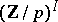for some indexing set, whereis cyclic of order). Then Serre's theorem asserts that there exist non-trivial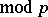cohomology classessuch that the product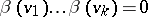, whereis the Bockstein operation associated to the exact coefficient sequence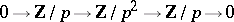(see [a9] and Cohomology operation). Note that for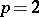this is simply the squaring operation.

For a finite-group, this can be made more explicit as follows. Each cohomology class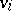corresponds to a (non-zero) homomorphism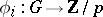and hence an index-subgroup. The class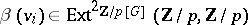can be represented as an extension class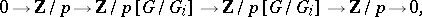wheredenotes the usual permutation module obtained by induction. When concatenated together, one obtains a representation of the product, which is an element in, as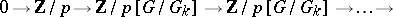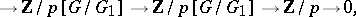which the theorem asserts to be the trivial extension class.

The original application of Serre's result was for proving that ifis a profinite group without elements of order, then the-cohomological dimension ofis equal to the-cohomological dimension offor any open subgroup(see [a8] for more on this; cf. also Cohomological dimension).

However, it is also a basic technical result used in proving the landmark result (see [a5] and [a6]) that the Krull dimension (cf. Dimension) of thecohomology of a finite groupis equal to the rank of the largest elementary Abelian-subgroup in. More precisely, Serre's theorem can be used to verify that for a finite non-Abelian-group, the Krull dimension of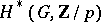(the maximal rank of a polynomial subalgebra) is determined on maximal proper subgroups, hence leading to an inductive argument which can be reduced to elementary Abelian subgroups.

This, in turn, can be extended to arbitrary finite groups and to cohomology with coefficients in a modular representation. Indeed, it is a basic result in the theory of complexity and cohomological varieties in representation theory. This is explained [a2], [a3] and [a4].